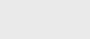4年前 (2020-02-09) |   抢沙发  1358

``````BigDecimal bd1 = new BigDecimal(0.1);
System.out.println("bd1="+bd1);
BigDecimal bd2 = new BigDecimal("0.1");
System.out.println("bd2="+bd2);
BigDecimal bd3 = BigDecimal.valueOf(0.1);
System.out.println("bd3="+bd3);``````

``````bd1=0.1000000000000000055511151231257827021181583404541015625
bd2=0.1
bd3=0.1``````

Effective Java》这本书里说过：

0.5能够表示，因为它可以表示成为1/2

0.75也能够表示，因为它可以表示成为1/2+1/(2^2)

0.875也能够表示，因为它可以表示成为1/2+1/(2^2)+1/(2^3)

``````System.out.println(0.5*3);
System.out.println(0.1*3);``````

``````BigDecimal bd4 = new BigDecimal("10");
BigDecimal bd5 = new BigDecimal("3");
System.out.println(bd4.divide(bd5));``````

``````Exception in thread "main" java.lang.ArithmeticException: Non-terminating decimal expansion; no exact representable decimal result.
at java.math.BigDecimal.divide(BigDecimal.java:1690)
at BigDecimalTest.main(BigDecimalTest.java:38)``````

``System.out.println(bd4.divide(bd5,2,BigDecimal.ROUND_DOWN));``

``````BigDecimal bd1 = new BigDecimal("0");
BigDecimal bd2 = new BigDecimal("0.0");
System.out.println(bd1.equals(bd2));
System.out.println(bd1.compareTo(bd2) == 0)``````

``````equals:false
compareTo:true``````### 注册